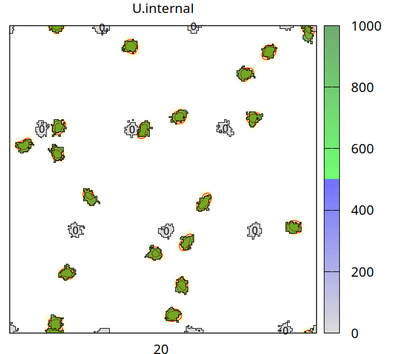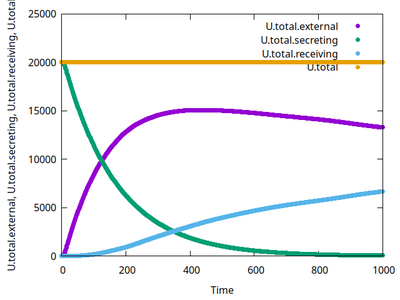# Mass-conserving secretion and uptake of a diffusible signal

## Introduction

The mass-conserving secretion and uptake of (signaling) molecules by cells can in Morpheus be achieved with Mappers and scaling fluxes by cell.volume. The intracellular amount of signal may trigger a change in a state variable that could downstream change cell behaviors.

This model, motivated by the user forum, shall demonstrate how such signaling processes can be coupled via a global field of diffusible molecules and how conservation of mass can be monitored over populations of cells.

## Description

There are two cell types (besides medium): cell_secreting (which secretes signal U with flux p into the Global/Field U.external) and cell_receiving (which takes U up from U.external with rate d and accumulates it in U.internal, when U.internal exceeds U.threshold=500 then cell_receiving changes its state U.superthreshold from 0 to 1). The chosen CPM settings let cells of different types repel each other and let cell_receiving chemotax upward the U.external gradient. The number (0 or 1) plotted in each receiving cell is the state of U.superthreshold.

Please also see the descriptions of related models like Autocrine chemotaxis and the cAMP dynamics during Dictyostelium aggregation. Moreover, the field U.external may get extra reaction terms, see this Example.Snapshot at time=20 showing the initial cell states (secreting cells in green and without any number, receiving cells in gray with the number 0).Snapshot at time=500 showing the concentration fields change (yellow/red colors in background with contour lines) and changed amounts of signaling molecules inside cells as given by the color bar.

The video shows how the secreting cells (initially green and without any number) lose the signal (corresponding to color bar), how the concentration fields change (yellow/red colors in background with contour lines) and receiving cells (with a number) take up the signal (change color from gray to blue and then green corresponding to color bar). When a threshold of internalized signal is crossed, then receiving cells change their state from 0 to 1 (shown as number inside receiving cells). Receiving cells chemotax upward in the gradient of the concentration field.Time courses of sums of signals in all secreting, receiving cells as well as the extracellular field show that the total amount is conserved.

## Model

Get this model via:

•  Download: DiffusibleSignal.xml
• XML Preview

<MorpheusModel version="4">
<Description>
<Title>Diffusible Signal</Title>
<Details>Full title:	Example for mass-conserving secretion and uptake of a diffusible signal
Contributors:L. Brusch
Date:	09.11.2023
Model ID:	https://identifiers.org/morpheus/M5491
For related models see
- Autocrine Chemotaxis https://identifiers.org/morpheus/M0031
- Dictyostelium https://identifiers.org/morpheus/M0034
</Details>
</Description>
<Global>
<Constant symbol="cell_density" value="0.0005"/>
<Field symbol="U.external" value="0">
<Diffusion rate="0.1"/>
</Field>
<System time-step="0.5" solver="Runge-Kutta [fixed, O(4)]">
<DiffEqn symbol-ref="U.external">
<Expression>p*U.internal - d*U.external</Expression>
</DiffEqn>
</System>
<Variable symbol="U.total.external" value="0"/>
<Mapper time-step="1.0" name="U.total.external">
<Input value="U.external"/>
<Output symbol-ref="U.total.external" mapping="sum"/>
</Mapper>
<Variable symbol="U.total.receiving" value="0"/>
<Mapper time-step="1.0" name="U.total.receiving">
<Input value="U.internal*(cell.type==celltype.cell_receiving.id)"/>
<Output symbol-ref="U.total.receiving" mapping="sum"/>
</Mapper>
<Variable symbol="U.total.secreting" value="0"/>
<Mapper time-step="1.0" name="U.total.secreting">
<Input value="U.internal*(cell.type==celltype.cell_secreting.id)"/>
<Output symbol-ref="U.total.secreting" mapping="sum"/>
</Mapper>
<Function symbol="U.total">
<Expression>U.total.external+U.total.secreting+U.total.receiving</Expression>
</Function>
</Global>
<Space>
<Lattice class="square">
<Size symbol="size" value="200, 200, 0"/>
<BoundaryConditions>
<Condition type="periodic" boundary="x"/>
<Condition type="periodic" boundary="y"/>
</BoundaryConditions>
<NodeLength value="1.0"/>
<Neighborhood>
<Distance>2.5</Distance>
</Neighborhood>
</Lattice>
<SpaceSymbol symbol="space"/>
</Space>
<Time>
<StartTime value="0"/>
<StopTime value="1000"/>
<RandomSeed value="0"/>
<TimeSymbol symbol="time"/>
</Time>
<CellTypes>
<CellType name="medium" class="medium">
<Constant symbol="p" name="production" value="0.0"/>
<Constant symbol="d" value="0.0"/>
<Property symbol="U.internal" value="0.0"/>
</CellType>
<CellType name="cell_secreting" class="biological">
<VolumeConstraint target="60" strength="1"/>
<SurfaceConstraint target="0.85" strength="1" mode="aspherity"/>
<Constant symbol="p" value="0.0001"/>
<Constant symbol="d" value="0.0"/>
<Property symbol="U.internal" value="1000.0"/>
<System time-step="0.1" solver="Runge-Kutta [fixed, O(4)]">
<DiffEqn symbol-ref="U.internal">
<Expression>-p*U.internal*cell.volume</Expression>
</DiffEqn>
</System>
</CellType>
<CellType name="cell_receiving" class="biological">
<VolumeConstraint target="60" strength="1"/>
<SurfaceConstraint target="0.85" strength="1" mode="aspherity"/>
<Chemotaxis strength="100" field="U.external"/>
<Constant symbol="p" value="0.0"/>
<Constant symbol="d" value="0.02"/>
<Constant symbol="U.threshold" value="500.0"/>
<Property symbol="U.uptake" value="0.0"/>
<Property symbol="U.internal" value="0.0"/>
<Property symbol="U.superthreshold" value="0.0"/>
<Mapper time-step="1.0" name="Uptake of U">
<Input value="d*U.external"/>
<Output symbol-ref="U.uptake" mapping="sum"/>
</Mapper>
<System time-step="0.1" solver="Runge-Kutta [fixed, O(4)]">
<DiffEqn symbol-ref="U.internal">
<Expression>U.uptake</Expression>
</DiffEqn>
<Rule symbol-ref="U.superthreshold">
<Expression>U.internal > U.threshold</Expression>
</Rule>
</System>
</CellType>
</CellTypes>
<CPM>
<Interaction default="0.0">
<Contact type2="medium" type1="cell_secreting" value="-10"/>
<Contact type2="medium" type1="cell_receiving" value="-10"/>
<Contact type2="cell_secreting" type1="cell_receiving" value="10"/>
</Interaction>
<MonteCarloSampler stepper="edgelist">
<MCSDuration value="1.0"/>
<Neighborhood>
<Order>2</Order>
</Neighborhood>
<MetropolisKinetics temperature="10.0"/>
</MonteCarloSampler>
<ShapeSurface scaling="norm">
<Neighborhood>
<Order>optimal</Order>
</Neighborhood>
</ShapeSurface>
</CPM>
<CellPopulations>
<Population type="cell_secreting" size="0">
<InitRectangle mode="random" number-of-cells="cell_density * size.x * size.y">
<Dimensions origin="0.0, 0.0, 0.0" size="size.x, size.y, 0"/>
</InitRectangle>
</Population>
<Population type="cell_receiving" size="0">
<InitRectangle mode="regular" number-of-cells="cell_density /2 * size.x * size.y">
<Dimensions origin="0.0, 0.0, 0.0" size="size.x, size.y, 0"/>
</InitRectangle>
</Population>
</CellPopulations>
<Analysis>
<Gnuplotter time-step="50" decorate="true">
<Terminal name="png" persist="true"/>
<Plot>
<Field surface="true" symbol-ref="U.external" max="5.0" isolines="5" min="0.0"/>
<Cells opacity="0.55" max="1000" min="0" value="U.internal">
<ColorMap>
<Color color="gray" value="0"/>
<Color color="blue" value="500"/>
<Color color="green" value="501"/>
<Color color="dark-green" value="1000"/>
</ColorMap>
</Cells>
<CellLabels value="U.superthreshold"/>
</Plot>
</Gnuplotter>
<ModelGraph include-tags="#untagged" format="svg" reduced="false"/>
<Logger time-step="1.0">
<Input/>
<Output>
<TextOutput/>
</Output>
<Plots>
<Plot time-step="-1">
<Style style="points"/>
<Terminal terminal="png"/>
<X-axis>
<Symbol symbol-ref="time"/>
</X-axis>
<Y-axis>
<Symbol symbol-ref="U.total.external"/>
<Symbol symbol-ref="U.total.secreting"/>
<Symbol symbol-ref="U.total.receiving"/>
<Symbol symbol-ref="U.total"/>
</Y-axis>
</Plot>
</Plots>
</Logger>
</Analysis>
</MorpheusModel>